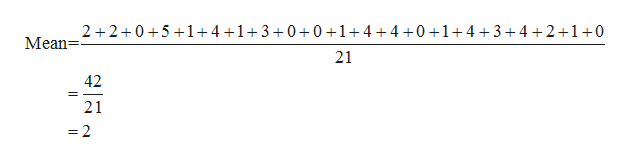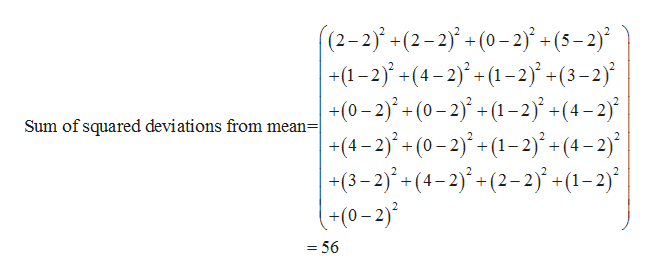# The question is, for the following scores find the mean, median, sum of squared deviations, variance, and standard deviation. 2,2,0,5,1,4,1,3,0,,0,1,4,,4,0,1,,4,3,4,2,1,0. Tnank you!

Question
1 views

The question is, for the following scores find the mean, median, sum of squared deviations, variance, and standard deviation. 2,2,0,5,1,4,1,3,0,,0,1,4,,4,0,1,,4,3,4,2,1,0. Tnank you!

check_circle

star
star
star
star
star
1 Rating
Step 1

The mean of the data is calculated as follows:help_outlineImage Transcriptionclose2 2 051+4 +1+3 +0+0 +1+4+4 +0 +1+ 4+3+4+2+1+0 21 Mean= 42 21 Il fullscreen
Step 2

Median is the middle most items. There are 21 items. Therefore, median is the 11th ((21+1)/2) item in the ordered arrangement (ascending or descending) of the data values.

The data in ascending order is as follows:

0,0,0,0,0,1,1,1,1,1,2,2,2,3,3,4,4,4,4,4,5.

Therefore, median is 2 (11th item in the ordered arrangement).

The sum of squared deviations is calculated as follows:help_outlineImage Transcriptionclose(2-2j+(2-2j- (0-2) + (5-2) | -+(1-2)(4-2)(1-2) (3-2 |+(0-2)+ (0-2) (1-2 +(4-2 |-+(4-2)-(0-2)+(1-2)+ (4-2) (3-2)j(4-2(2-2)j +(1-2) (+0-2) Sum of squared deviations from mean| _ = 56 fullscreen
Step 3

The sample variance is calculated...

### Want to see the full answer?

See Solution

#### Want to see this answer and more?

Solutions are written by subject experts who are available 24/7. Questions are typically answered within 1 hour.*

See Solution
*Response times may vary by subject and question.
Tagged in

### Other